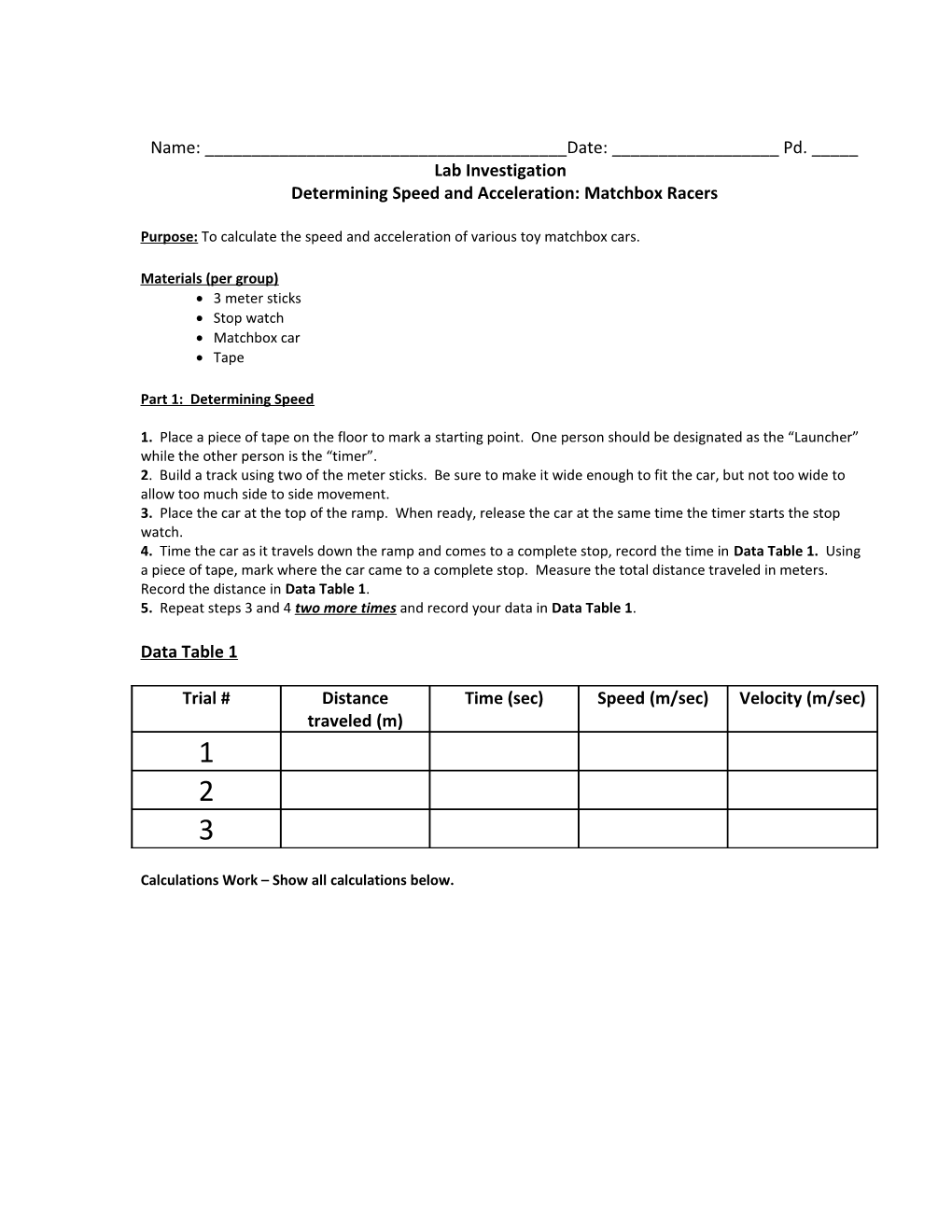# Determining Speed and Acceleration: Matchbox RacersName: ______Date: ______Pd. _____

Lab Investigation

Determining Speed and Acceleration: Matchbox Racers

Purpose: To calculate the speed and acceleration of various toy matchbox cars.

Materials (per group)

• 3 meter sticks
• Stop watch
• Matchbox car
• Tape

Part 1: Determining Speed

1. Place a piece of tape on the floor to mark a starting point. One person should be designated as the “Launcher” while the other person is the “timer”.

2. Build a track using two of the meter sticks. Be sure to make it wide enough to fit the car, but not too wide to allow too much side to side movement.

3. Place the car at the top of the ramp. When ready, release the car at the same time the timer starts the stop watch.

4. Time the car as it travels down the ramp and comes to a complete stop, record the time in Data Table 1. Using a piece of tape, mark where the car came to a complete stop. Measure the total distance traveled in meters. Record the distance in Data Table 1.

5. Repeat steps 3 and 4 two more times and record your data in Data Table 1.

Data Table 1

Trial # / Distance traveled (m) / Time (sec) / Speed (m/sec) / Velocity (m/sec)
1
2
3

Calculations Work – Show all calculations below.

Part 2: Determining Acceleration

1. Place two pieces of tape somewhere in the middle of the “track” but spaced a fair distance apart. (Keep in mind the distance the car traveled in Part 1 while setting this up).

2. You will need one “launcher” and two “timers” for this test. One timer will record the time it takes the car to travel from the first piece of tape to the second piece of tape, the second timer will record the time it takes the car to travel from the first piece of tape to a complete stop.

3. Measure the distance between the two pieces of tape (Distance 1). Record the distance in Data table 2. Measure the distance (Distance 2) from tape 1 to complete stop in Data Table 2.

4. Record the time from Tape 1 to Tape 2 (Time 1) in Data Table 2. Record the time from Tape 1 to complete stop (Time Total ) in Data Table 2.

Data Table 2

Distance 1 (m) / Time 1 (sec) / Calculated velocity (i)
D1/T1 / Calculated velocity (f)
D2/total time / Distance 2 (m) / Total time (sec)

Velocity Calculations: Show all your work for your Vi and Vf calculations below.

Calculate the acceleration of the car below: Show all your work for your acceleration calculations below.

Part 3: Analysis Questions: Answer each of the following questions in complete sentences.

1. In part 1 of the experiment you calculated the speed of a toy car. Were you calculating constant speed, instantaneous speed or average speed? Explain your answer.

2. How would the velocity reading differ if you repeated the experiment in exactly the same way in the opposite direction?

3. Why is your acceleration value in part two of the experiment a negative value?

4. What would the initial velocity of the car be in miles/hour? Show your calculations below.

5. What force(s) acted on your car to bring it to a stop? (Be Specific)

6. Write a 5 sentence paragraph conclusion. Be sure to write a sentence to address the following things: What was the purpose of your lab? What were your results? Why do you think you got the results you did? What were some sources of error I the experiment? What are some improvements you could make to the lab for the future?